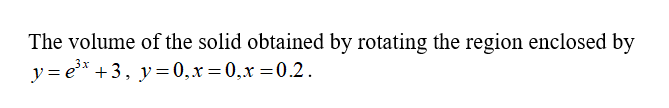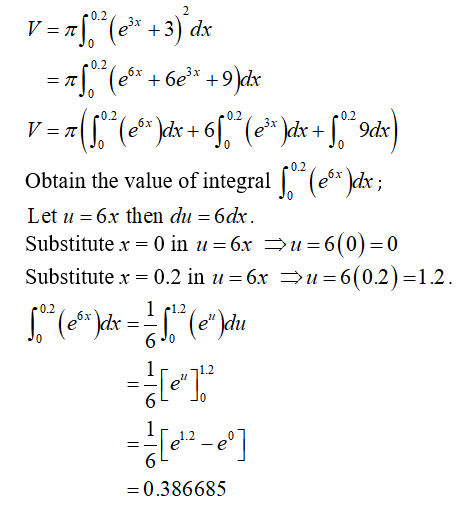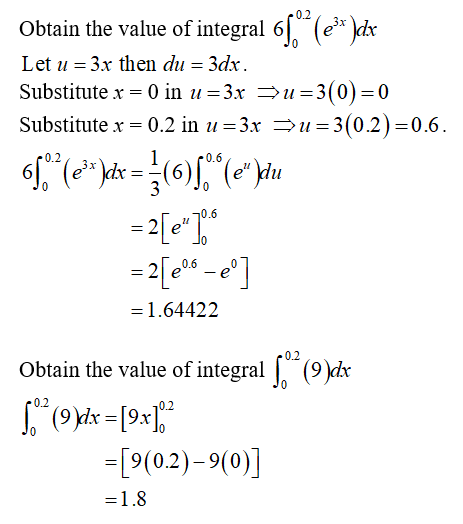# Problem 8 The volume of the solid obtained by rotating the region enclosed byy=(e^3x)+3, y=0, x=0, x=0.2 about the x-axis can be computed using the method of disksor washers via an integralV=∫[upper limit=b, lower limit=a]______ dxwith limits of integration a=______ and b=_______

Question
2 views

Problem 8

The volume of the solid obtained by rotating the region enclosed by

y=(e^3x)+3, y=0, x=0, x=0.2

about the x-axis can be computed using the method of disksor washers via an integral

V=∫[upper limit=b, lower limit=a]______ dx

with limits of integration a=______ and b=_______

check_circle

Given information:Find the volume of solid:Step 3...

### Want to see the full answer?

See Solution

#### Want to see this answer and more?

Solutions are written by subject experts who are available 24/7. Questions are typically answered within 1 hour.*

See Solution
*Response times may vary by subject and question.
Tagged in